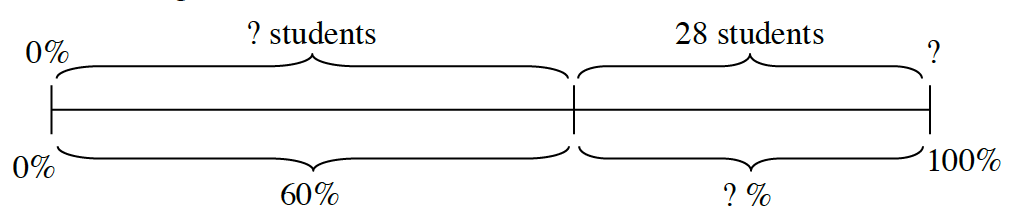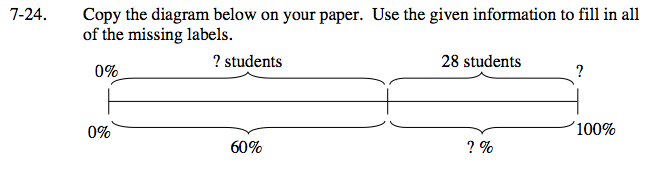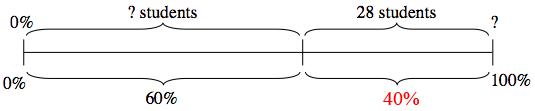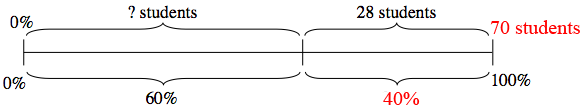Home > CC2MN > Chapter cc27 > Lesson cc27.1.2 > Problem7-24

7-24.
1. Copy the diagram below on your paper. Use the given information to fill in all of the missing labels. Homework Help ✎

2.First solve for the lower half of the diagram.

Since the entire diagram is representative of 100% of the students, and the first portion represents 60%, the second portion must represent 40%.28 students is 40% of how many total students?

To find the remaining number of students for the first portion, subtract 28 from the total number of students.

$\frac{40}{100}=\frac{28}{x}$